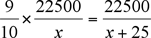Name: bidhu Who is asking: Other Level of the question: All Question: A reduction of 10% in the price of tea enables a dealer to purchase 25 kg more tea for Rs 22500.What is the reduced price per kg of tea?What was the original price per kg? Let us say that dealer originally paid Rs 22500 for x kg of tea.  That means each kg of tea was costing him Rs 22500/x. After the price reduction, the dealer pays Rs 22500 for (x+25) kg of tea.  So the new cost per kg is Rs 22500/(x+25). Since you are told that the reduction is 10%, you know that 90% of the original price is the new price.  So 90% (9/10)of the original cost equals the reduced cost. Let's put all this together:Now you can re-arrange this equation to solve for x, the original amount of tea the dealer could get for Rs 22500. Once you have the original amount of tea, you can insert that value into the fractions to get the price per kg before and after the price reduction. I hope this helps you. Stephen La Rocque>